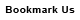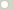# gram-force centimeter (Torque, Metric)

## 1 gram-force centimeter is equal to:Metric newton meter 9.80665012480924e-05 newton centimeter 0.00980665012480924 dyne meter 9.80665012480924 dyne centimeter 980.665012480924 kilogram-force meter 1e-05 kilogram-force centimeter 0.001 gram-force meter 0.01 British and U.S. long ton-force foot 3.229024e-08 short ton-force foot 3.6165069e-08 pound-force foot 7.2330139e-05 pound-force inch 0.00086796166 ounce-force inch 0.013887387ConversionsAreaCapacity and VolumeCircular measureComputer storageCurrencyDistance and LengthEnergy and WorkFuel ConsumptionMass and WeightPowerPressureSpeedTemperatureTimeTorqueSearchLink to us